HT for Web 不止自主研发了强大的基于 HTML5 的 2D、3D 渲染引擎，为可视化提供了丰富的展示效果。介于 2D 组态和 3D 组态上，Hightopo（以下简称 HT ）的 HT for Web 产品上的有着丰富的组态化可供选择，本文将介绍如何运用 HT 丰富的 2/3D 组态搭建出一个隧道监控可视化系统的解决方案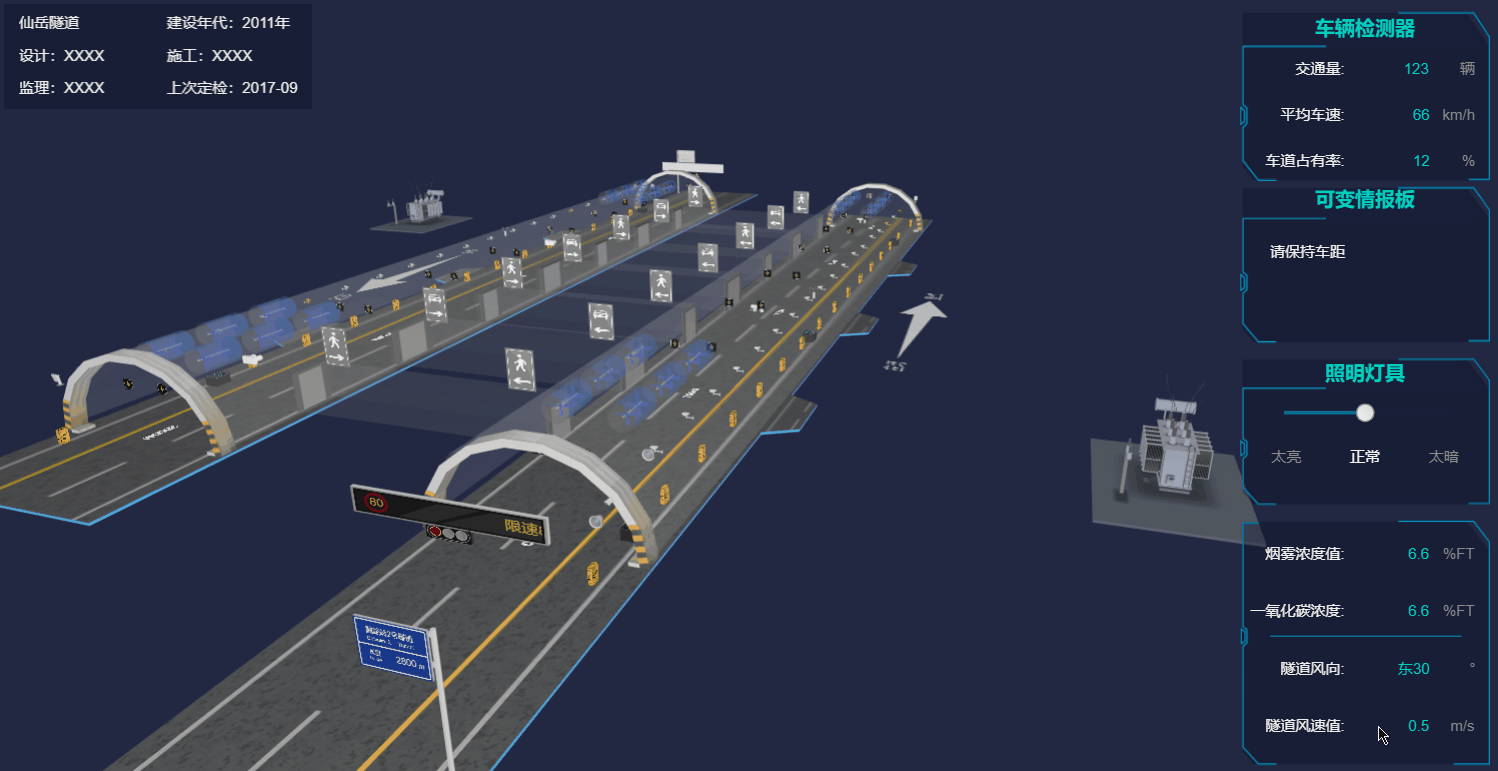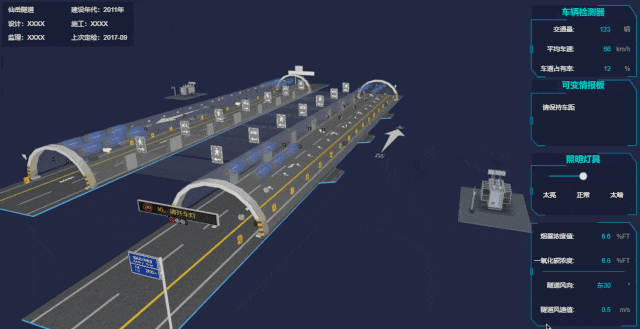```// 数据容器
dm = new ht.DataModel();
// 3d 场景
g3d = new ht.graph3d.Graph3dView(dm);
// 将场景添加到 body 中

```addToDOM = function(){
var self = this,
// 获取组件的底层 div
view = self.getView(),
style = view.style;
// 将组件底层div添加进body中
document.body.appendChild(view);
// ht 默认将所有的组件的position都设置为absolute绝对定位
style.left = '0';
style.right = '0';
style.top = '0';
style.bottom = '0';
// 窗口大小改变事件，调用刷新函数
window.addEventListener('resize', function () { self.iv(); }, false);
}```

```// xhrLoad 函数是一个异步加载文件的函数
// 将 json 文件中的文本转为我们需要的 json 格式的内容
var json = ht.Default.parse(text);
// 反序列化数据容器，解析用于生成对应的Data对象并添加到数据容器 这里相当于把 json 文件中生成的 ht.Node 节点反序列化到数据容器中，这样数据容器中就有这个节点了
dm.deserialize(json);
});```

```dm.each(function(data) {
// 对“电话”进行批量
if (data.s('front.image') === 'assets/sos电话.png'){
data.s('batch', 'sosBatch');
}
// 逃生通道批量（透明度也会影响性能）
else if (data.s('all.color') === 'rgba(222,222,222,0.18)') {
data.s('batch', 'emergencyBatch');
}
else if (data.s('shape3d') === 'models/隧道/摄像头.json' || data.s('shape3d') === 'models/隧道/横洞.json' || data.s('shape3d') === 'models/隧道/卷帘门.json') {
// 个别摄像头染色了 不做批量
if(!data.s('shape3d.blend'))
// 基础批量什么也不做
data.s('batch', 'basicBatch');
}
else if (data.s('shape3d') === 'models/大型变压器/变压器.json') {
data.s('batch', 'tileBatch');
data.setToolTip('单击漫游，双击车祸地点出现图标');
}
else if (data.getDisplayName() === '地面') {
// 设置隧道“地面”不可选中
data.s('3d.selectable', false);
}
else if (data.s('shape3d') === 'models/隧道/排风.json') {
// 排风扇的模型比较复杂，所以做批量
data.s('batch', 'fanBatch');
}
else if (data.getDisplayName() === 'arrow') {
// 隧道两旁的箭头路标
if (data.getTag() === 'arrowLeft') data.s('shape3d.image', 'displays/abc.png');
else data.s('shape3d.image', 'displays/abc2.png');
data.s({
'shape3d': 'billboard',
// 缓存，设置了 cache 的代价是需要设置 invalidateShape3dCachedImage
'shape3d.image.cache': true,
// 设置这个值，图片上的锯齿就不会太明显了（若图片类型为 json，则设置 shape3d.dynamic.transparent)
'shape3d.transparent': true
});
g3d.invalidateShape3dCachedImage(data);
}
// 隧道入口处的情报板
else if (data.getTag() === 'board' || data.getTag() === 'board1') {
// 业务属性，用来控制文本的位置[x,y,width,height]
data.a('textRect', [0, 2, 244, 46]);
// 业务属性，设置文本内容
data.a('limitText', '限速80，请开车灯');
var min = -245;
var name = 'board' + data.getId();
window[name] = setInterval(function() {
// 设置情报板中的文字向左滚动，并且当文字全部显示时重复闪烁三次
circleFunc(data, window[name], min);
}, 100);
}

//给逃生通道上方的指示板 动态设置颜色
var infos = ['人行横洞1', '人行横洞2', '人行横洞3', '人行横洞4', '车行横洞1', '车行横洞2', '车行横洞3'];
infos.forEach(function(info) {
if(data.getDisplayName() === info) {
data.a('emergencyColor', 'rgb(138, 138, 138)');
}
});

infos = ['车道指示器', '车道指示器1', '车道指示器2', '车道指示器3'];
infos.forEach(function(info) {
if (data.getDisplayName() === info) {
// 考虑到性能问题 将六面体变换为 billboard 类型元素
createBillboard(data, 'assets/车道信号-过.png', 'assets/车道信号-过.png', info);
}
});
});```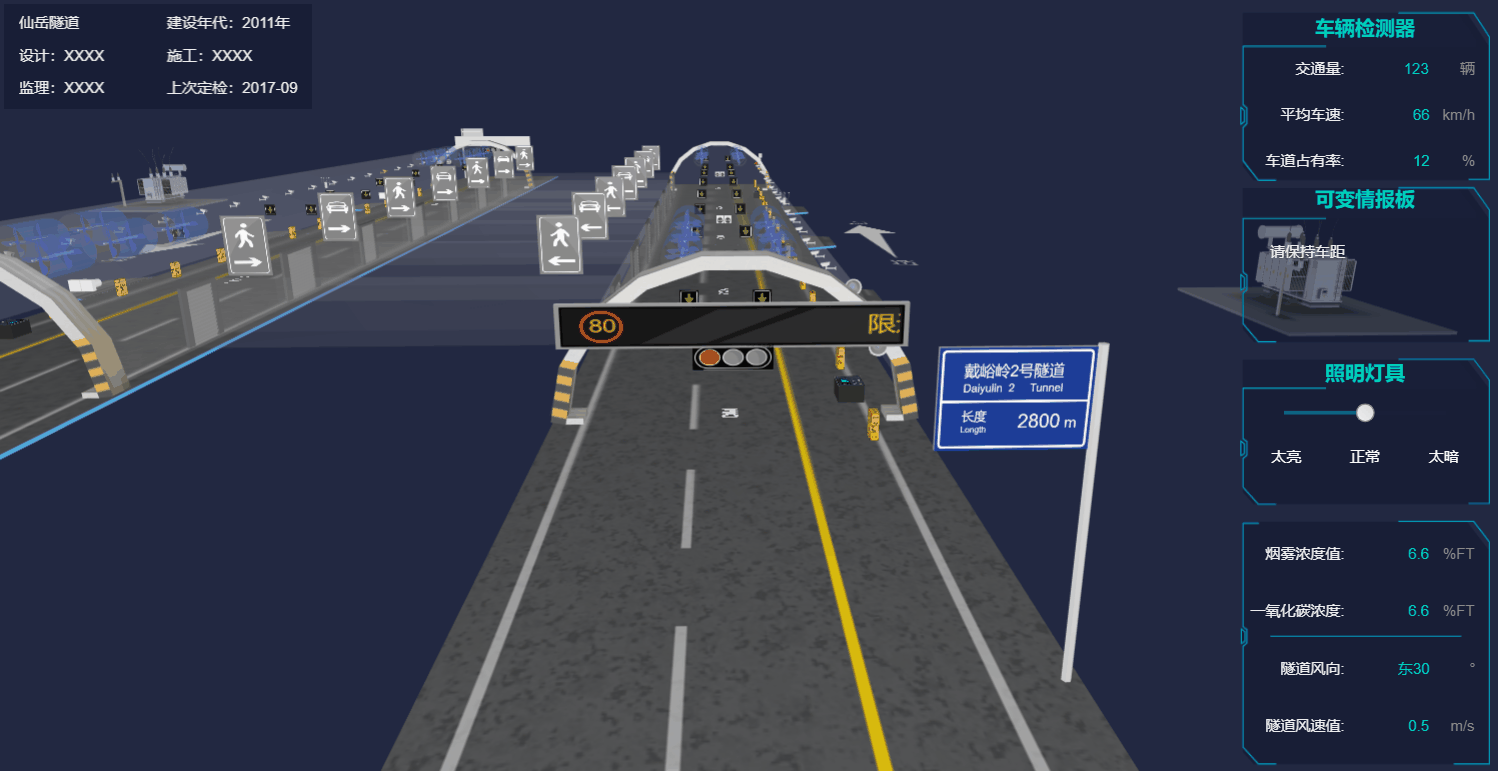```// 设置情报板中的文字向左滚动，并且当文字全部显示时重复闪烁三次
function circleFunc(data, timer, min) {
// 获取当前业务属性 limitText 的内容
var text = data.a('limitText');
// 设置业务属性 textRect 文本框的坐标和大小
data.a('textRect', [data.a('textRect')-5, 2, 244, 46]);
if (parseInt(data.a('textRect')) <= parseInt(min)) {
data.a('textRect', [255, 2, 244, 46]);
}
else if (data.a('textRect') === 0) {
clearInterval(timer);
var index = 0;
// 设置多个 timer 是因为能够进入这个函数中的不止一个 data，如果在同一时间多个 data 设置同一个 timer，那肯定只会对最后一个节点进行动画。后面还有很多这种陷阱，要注意
var testName = 'testTimer' + data.getId();
window[testName] = setInterval(function() {
index++;
// 如果情报板中文本内容为空
if(data.a('limitText') === '') {
setTimeout(function() {
// 设置为传入的 text 值
data.a('limitText', text);
}, 100);
}
else {
setTimeout(function() {
// 若情报板中的文本内容不为空，则设置为空
data.a('limitText', '');
}, 100);
}
// 重复三次
if(index === 11) {
clearInterval(window[testName]);
data.a('limitText', text);
}
}, 100);

setTimeout(function() {
timer = setInterval(function() {
// 回调函数
circleFunc(data, timer, min);
}, 100);
}, 1500);
}
} ``````node.s({
'shape3d': 'billboard',
'shape3d.image': imageUrl,
'shape3d.image.cache': true
});
// 还记得用 shape3d.image.cache 的代价么？
g3d.invalidateShape3dCachedImage(node); ```

Camera 缓慢偏移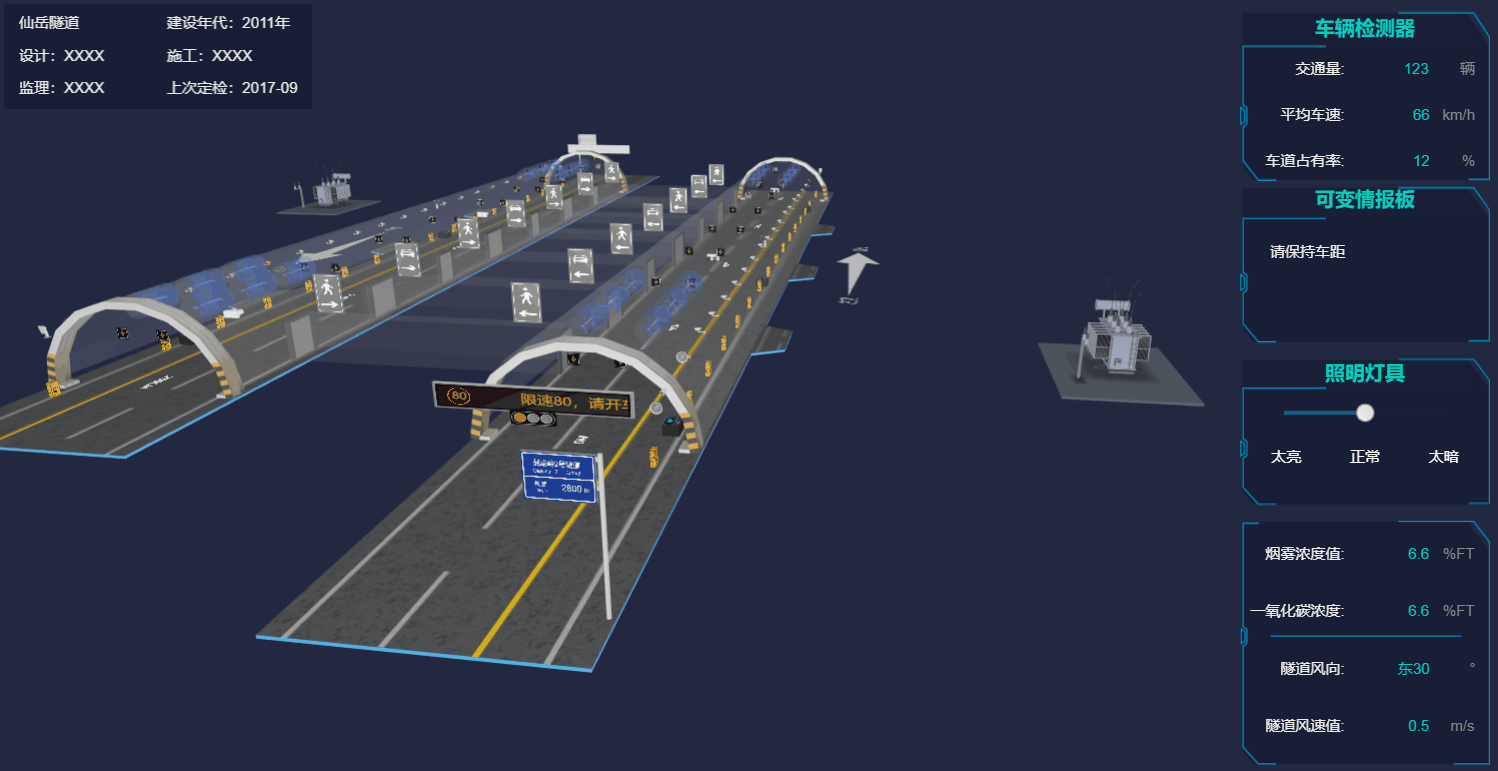```// 设置“目标”位置
function setCenter(center, finish) {
// 获取当前“目标”位置，为一个数组，而 getCenter 数组会在视线移动的过程中不断变化，所以我们先拷贝一份
var c = g3d.getCenter().slice(0),
// 当前x轴位置和目标位置的差值
dx = center - c,
dy = center - c,
dz = center - c;
// 启动 500 毫秒的动画过度
ht.Default.startAnim({
duration: 500,
action: function(v, t) {
// 将“目标”位置缓慢从当前位置移动到设置的位置处
g3d.setCenter([
c + dx * v,
c + dy * v,
c + dz * v
]);
}
});
};

// 设置“眼睛”位置
function setEye(eye, finish) {
// 获取当前“眼睛”位置，为一个数组，而 getEye 数组会在视线移动的过程中不断变化，所以我们先拷贝一份
var e = g3d.getEye().slice(0),
dx = eye - e,
dy = eye - e,
dz = eye - e;
// 启动 500 毫秒的动画过度
ht.Default.startAnim({
duration: 500,
// 将 Camera 位置缓慢地从当前位置移动到设置的位置
action: function(v, t) {
g3d.setEye([
e + dx * v,
e + dy * v,
e + dz * v
]);
}
});
};```

```// 获取事件对象的三维坐标
var p3 = e.data.p3(),
// 获取事件对象的三维尺寸
s3 = e.data.s3(),
// 获取事件对象的三维旋转值
r3 = e.data.r3();
// 设置“目标”位置为当前事件对象的三维坐标值
setCenter(p3);
// 如果节点的 y 轴旋转值 不为 0
if (r3 !== 0) {
// 浮点负数得做转换才能进行比值
if (parseFloat(r3.toFixed(5)) === parseFloat(-3.14159)) {
// 设置camera 的目标位置
setEye([p3, p3+s3, p3 * Math.abs(r3*2.3/6)]);
}
else if (parseFloat(r3.toFixed(4)) === parseFloat(-1.5708)) {
setEye([p3 * Math.abs(r3/1.8), p3+s3, p3]);
}
else {
setEye([p3 *r3, p3+s3, p3]);
}
}
else {
setEye([p3, p3+s3*2, p3+1000]);
}```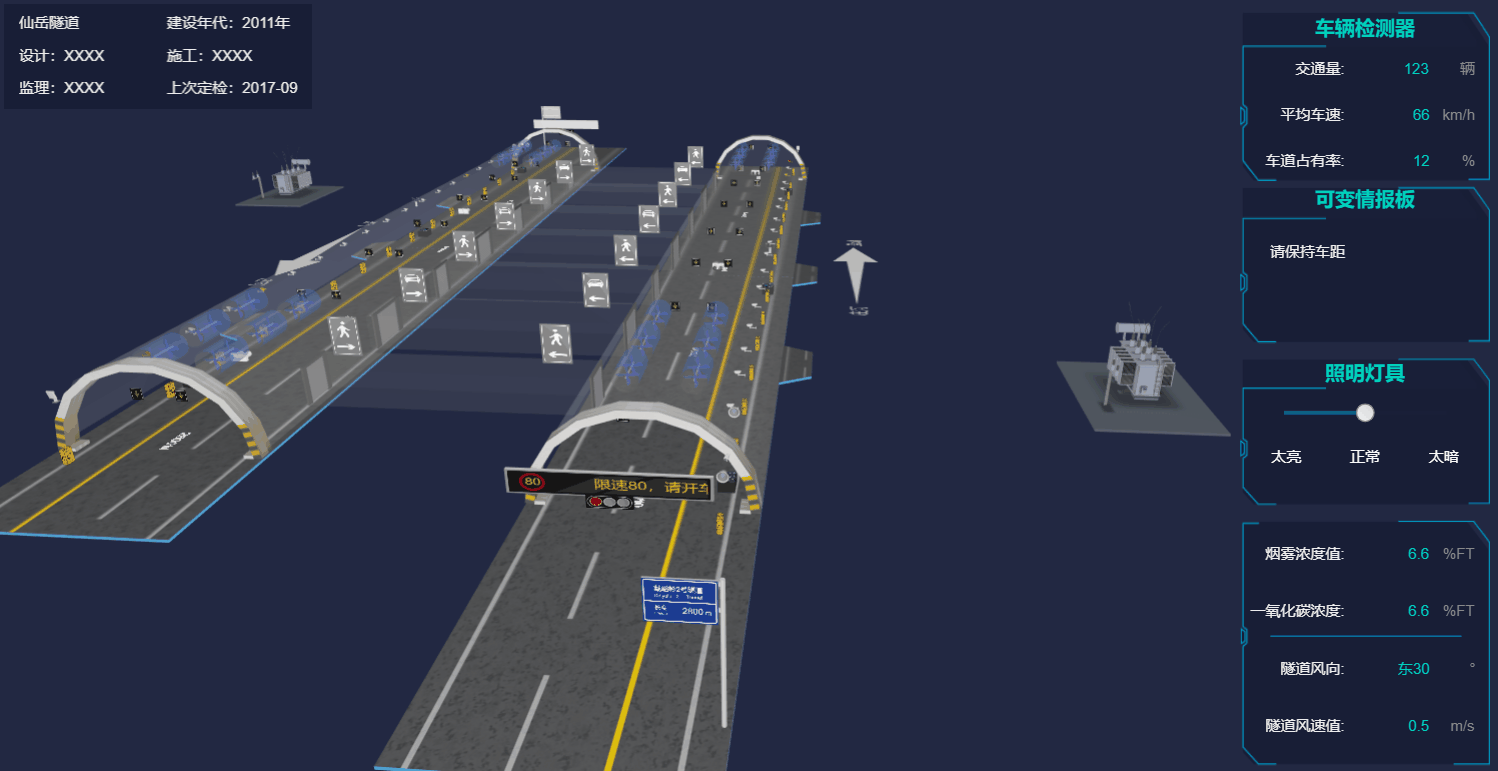```g3d.addInteractorListener(function(e) {
if(e.kind === 'doubleClickData') {
// 有“事故”图标节点存在
if (e.data.getTag() === 'jam') return;
// 如果双击对象是变压器
if (e.data.s('shape3d') === 'models/大型变压器/变压器.json') {
index++;
// 通过唯一标识 tag 标签获取“事故”图标节点对象
var jam = dm.getDataByTag('jam');
if(index === 1){
var jam = dm.getDataByTag('jam');
jam.s({
// 设置节点在 3d 上可见
'3d.visible': true,
// 设置节点为 billboard 类型
'shape3d': 'billboard',
// 设置 billboard 的显示图片
'shape3d.image': 'assets/车祸.png',
// 设置 billboard 图片是否缓存
'shape3d.image.cache': true,
// 是否始终面向镜头
'shape3d.autorotate': true,
// 默认保持图片原本大小，设置为数组模式则可以设置图片显示在界面上的大小
'shape3d.fixSizeOnScreen': [30, 30],
});
// cache 的代价是节点需要设置这个函数
g3d.invalidateShape3dCachedImage(jam);
}
else {
jam.s({
// 第二次双击变压器就将所有一切恢复“事故”之前的状态
'3d.visible': false
});
dm.each(function(data) {
var p3 = data.p3();
if ((p3 < jam.p3()) && data.getDisplayName() === '车道指示器1') {
data.s('shape3d.image', 'assets/车道信号-过.png');
}
if(data.getTag() === 'board1') {
data.a('limitText', '限速80，请开车灯');
}
});
index = 0;
}
}
}
});```

```// 点击图元
else if (e.kind === 'clickData'){
timer = setTimeout(function() {
clearTimeout(timer);
// 如果是“事故”图标节点
if (e.data.getTag() === 'jam') {
// 创建一个对话框
createDialog(e.data);
}
}, 200);
}``````// 弹出框右边的表单
function createForm4(node, dialog) {
// 表单组件
var form = new ht.widget.FormPane();
// 设置表单组件的宽
form.setWidth(200);
// 设置表单组件的高
form.setHeight(200);
// 获取表单组件的底层 div
var view = form.getView();
// 将表单组件添加到 body 中
document.body.appendChild(view);

var infos = [
'编辑框内容为：2辆',
'编辑框内容为：客车-客车',
'编辑框内容为：无起火',
'编辑框内容为：超车道'
];
infos.forEach(function(info) {
// 向表单中添加行
info
// 第二个参数为行宽度，小于1的值为相对值
], [0.1]);
});

{
// 添加一行的“确认”按钮
button: {
label: '确认',
// 按钮点击事件触发
onClicked: function() {
// 隐藏对话框
dialog.hide();
dm.each(function(data) {
var p3 = data.p3();
// 改变“车道指示器”的显示图片为红色×，这里我是根据“事故”图标节点的坐标来判断“车道显示器”是在前还是在后的
if ((p3 < node.p3()) && data.getDisplayName() === '车道指示器1') {
data.s('shape3d.image', 'assets/车道信号-禁止.png');
}
// 将隧道口的情报板上的文字替换
if(data.getTag() === 'board1') {
data.a('limitText', '超车道两车追尾，请减速慢行');
}
});
}
}
}
], [0.1]);
return form;
}```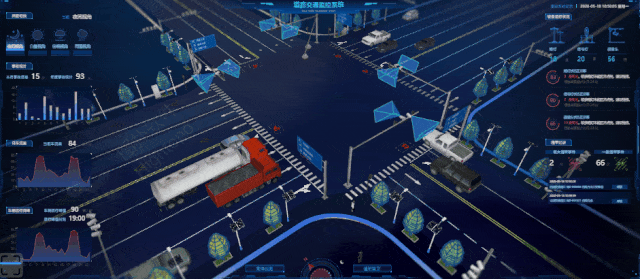2019 我们也更新了数百个工业互联网 2D/3D 可视化案例集，在这里你能发现许多新奇的实例，也能发掘出不一样的工业互联网：https://mp.weixin.qq.com/s/ZbhB6LO2kBRPrRIfHlKGQA